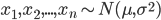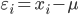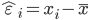# thesIt

##Arif

• #### 12:19:08 pm on August 22, 2010 | # | 0 Tags: basic, error, residual error, statistical error, wikipedia

If we assume a normally distributed population with mean μ and standard deviation σ, and take sample$x_{1}, x_{2},..., x_{n}\sim N(\mu,\sigma^{2})$

statistical error is then$\varepsilon_{i}=x_{i}-\mu$

## Residual

while residual is$\hat{\varepsilon}_{i}=x_{i}-\bar{x}$

hat over the letter ε indicates an observable estimate of an unobservable quantity called ε.